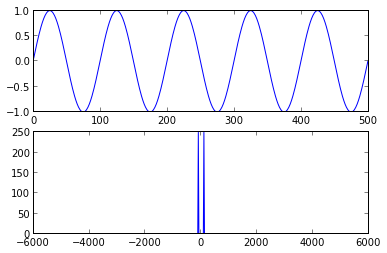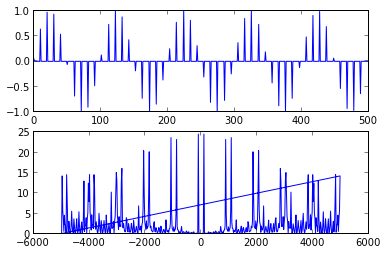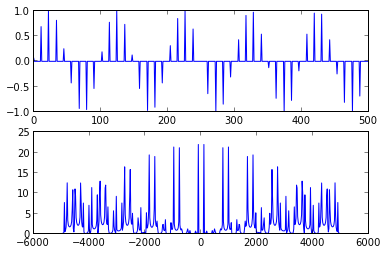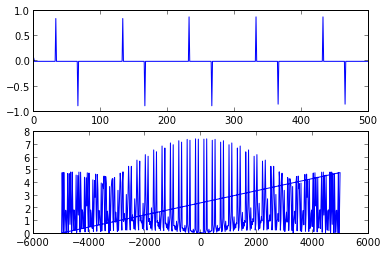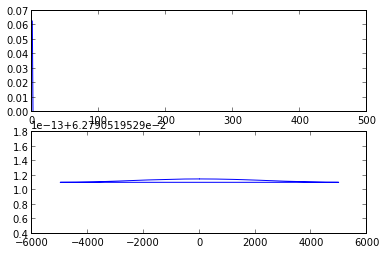In :
import scipy.signal

def plotspec(x, Ts):
fig = figure()
ax1.plot(x)

q = fft.fft(x)
ax2.plot(fft.fftfreq(len(x), Ts), abs(q))

In :
# sine100hzsamp.m : Simulated sampling of the 100Hz sine wave

f=100
time=0.05
Ts=1.0/10000.0
t = linspace(0, time, time/Ts)

w = sin(2*pi*f*t)

ss = 10

x = linspace(0, len(w)-1, len(w)/ss).astype(int)

wk = w.take(x)
ws = zeros(len(w))
ws.put(x,wk)

plot(t,w)
plot(t,ws,'r')

Out:
[<matplotlib.lines.Line2D at 0x10cabf090>]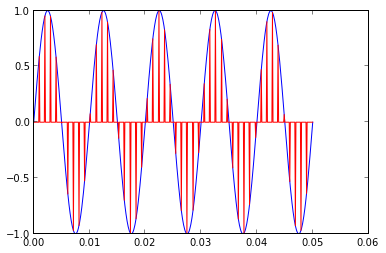6.8. Modify sine100hzsamp.m to create an oversampled sinc wave, then sample this with ss=10. Repeate with 30,100,200. Comment on what's happening.

In :
f=100
time=0.05
Ts=1.0/10000.0
t = linspace(0, time, time/Ts)

w = sinc(2*pi*f*t)

plotspec(w, Ts)

for ss in [10,30,100,200]:
x = linspace(0, len(w)-1, len(w)/ss).astype(int)

wk = w.take(x)
ws = zeros(len(w))
ws.put(x,wk)

plotspec(ws, Ts)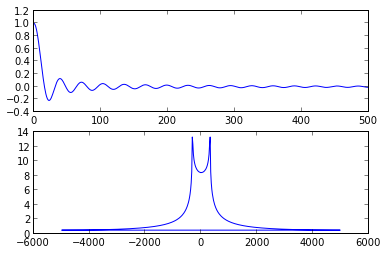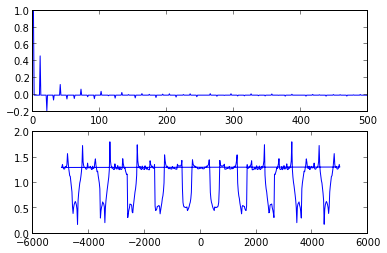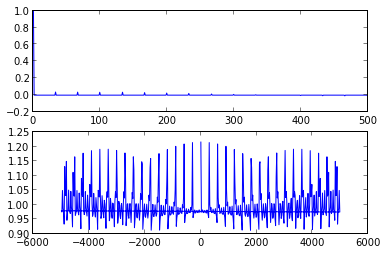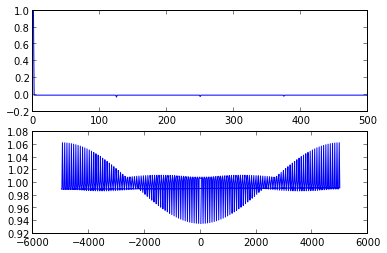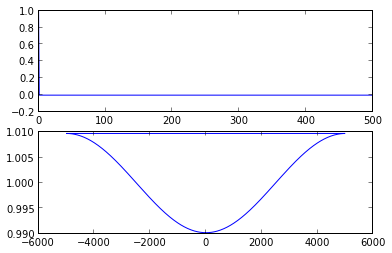6.9. Plot hte spectrum of the 100Hz sine wave when it's downsampled by 10,11,30, and 200.

In :
f=100
time=0.05
Ts=1.0/10000.0
t = linspace(Ts, time, time/Ts)

w = sin(2*pi*f*t)

plotspec(w, Ts)

for ss in [10,11,30,200]:

x = linspace(0, len(w)-1, len(w)/ss).astype(int)

wk = w.take(x)
ws = zeros(len(w))
ws.put(x,wk)

plotspec(ws, Ts)